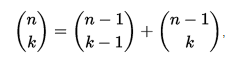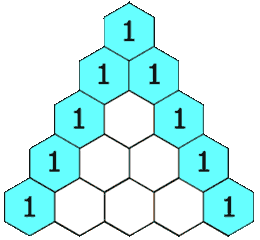# Pascal's Triagle Basic Information

suggest change

One of the most interesting Number Patterns is Pascal’s Triangle. The Name “Pascal’s Triangle” named after Blaise Pascal, a famous French Mathematician and Philosopher.

In Mathematics, Pascal’s Triangle is a triangular array of binomial coefficients.The rows of Pascal’s triangle are conventionally enumerated starting with row n = 0 at the top (the 0th row). The entries in each row are numbered from the left beginning with k = 0 and are usually staggered relative to the numbers in the adjacent rows.

The triangle is constructed in the below manner:

• In the topmost row, there is a unique nonzero entry 1.
• Each entry of each subsequent row is constructed by adding the number above and to the left with the number above and to the right, treating blank entries as 0.

For example, the initial number in the first (or any other) row is 1 (the sum of 0 and 1), whereas the numbers 1 and 3 in the third row are added to produce the number 4 in the fourth row.

Equation to generate each entry in Pascal triangle:for any non-negative integer n and any integer k between 0 and n, inclusive. This recurrence for the binomial coefficients is known as Pascal’s rule. Pascal’s triangle has higher dimensional generalizations. The three-dimensional version is called Pascal’s pyramid or Pascal’s tetrahedron, while the general versions are called Pascal’s simplices.

Example of Pascal’s Triangle: